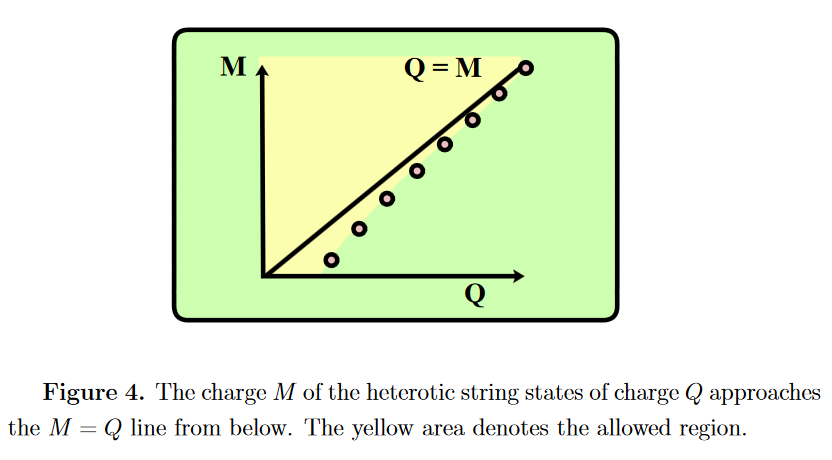# nLab weak gravity conjecture

Contents

### Context

#### Gravity

gravity, supergravity

# Contents

## Idea

The weak gravity conjecture is a conjecture (Arkani-Hamed, Motl, Nicolis, Vafa 06) often summarized as saying that for gravity or quantum gravity to be consistent, the force of the field gravity must be weaker than that of any of the gauge field forces, in suitable units.

## Loose statement

More precisely, what is argued in (AH-M-N-V 06, section 2) is that there alwayst must be “elementary particles” for which the ratio $m/{\vert q\vert}$ of their mass over their gauge field charge (e.g. electric charge, magnetic charge) is smaller than one: $m/{\vert q\vert} \lt 1$ (in natural units).

The argument for this in (AH-M-N-V 06, section 2.2) is the following. The extremal (BPS state) bound for charged black holes is that their mass $M$ exceeds or equals their charge ${\vert Q\vert}$, $M/{\vert Q\vert} \geq 1$. Now via black hole radiation any black hole is supposed to eventually decay by radiating away elementary particle quanta. If there were no elementary particle with $m/{\vert q\vert} \leq 1$, so the argument goes, then by radiating away particles with $m/{\vert q\vert} \gt 1$ the black hole would eventually lose all its mass, while still having some non-vanishing charge left. But such “black hole remnants” have been argued to lead to inconsistency (Susskind 96)

## In string theory

The weak gravity conjecture was originally motivated from string theory, which is argued to validate the “weak gravity” assumption.

In (AH-M-N-V 06, section 4) it is argued that the closed superstring excitattion states in heterotic string theory KK-compactified on to 4d satisfy the condition $m/{\vert q\vert} \lt 1$:## Relation to cosmic censorship

In 2015 Cumrun Vafa has argued that the weak gravity conjecture implies the cosmic censorship hypothesis: The latter turns out to be generally false, even in in 4 dimensions (Crisford-Santos 17), but there are arguments that configurations violating it also violate the assumption of “weak gravity”.

Subsequent calculations by Santos and Crisford supported Vafa’s hunch; the simulations they’re running now could verify that naked singularities become cloaked in black holes right at the point where gravity becomes the weakest force. (Wolchover, June 20 2017)

For more on this see Horowitz-Santos 19

The statement is due to

with reference to arguments in

Review is in

• Eran Palti, The Swampland: Introduction and Review, lecture notes (arXiv:1903.06239)

Further discussion includes

Relation to cosmic censorship hypothesis

Relation, via AdS/CFT and the conformal bootstrap, to the solution of the sphere packing problem in dimensions 8 and 24: